U.S. Department of Transportation
1200 New Jersey Avenue, SE
Washington, DC 20590
202-366-4000

Federal Highway Administration Research and Technology
Coordinating, Developing, and Delivering Highway Transportation InnovationsThis report is an archived publication and may contain dated technical, contact, and link information
 Federal Highway Administration > Publications > Research Publications > 04100 > Safety Effects of Marked Versus Unmarked Crosswalks at Uncontrolled Locations Final Report and Recommended Guidelines
Publication Number: FHWA-HRT-04-100
Date: September 2005

# Safety Effects of Marked Versus Unmarked Crosswalks at Uncontrolled Locations Final Report and Recommended Guidelines

PDF files can be viewed with the Acrobat® Reader®

## APPENDIX C. PLOTS OF EXPECTED PEDESTRIAN CRASHES BASED ON THE FINAL NEGATIVE BINOMIAL PREDICTION MODEL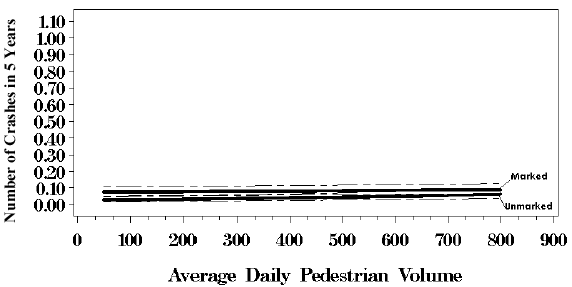Figure 45. Response curves with 95 percent confidence intervals based on negative binomial regression model, two lanes with no median, average daily motor vehicle traffic = 10,000.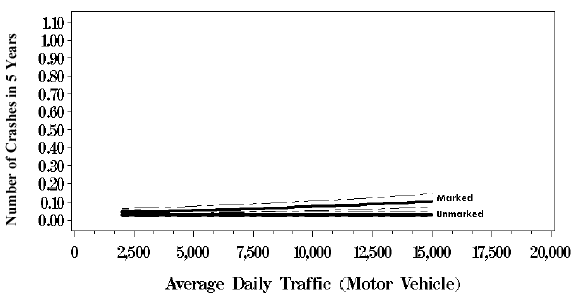Figure 46. Response curves with 95 percent confidence intervals based on negative binomial regression model, two lanes with no median, average daily pedestrian volume = 100.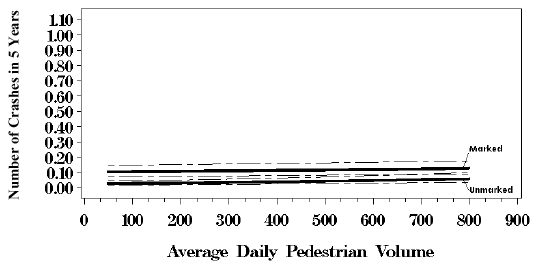Figure 47. Response curves with 95 percent confidence intervals based on negative binomial regression model, two lanes with no median, average daily motor vehicle traffic = 15,000.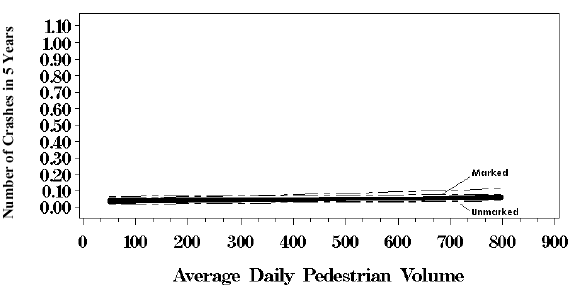Figure 48. Response curves with 95 percent confidence intervals based on negative binomial regression model, two lanes with no median, average daily motor vehicle traffic = 2,000.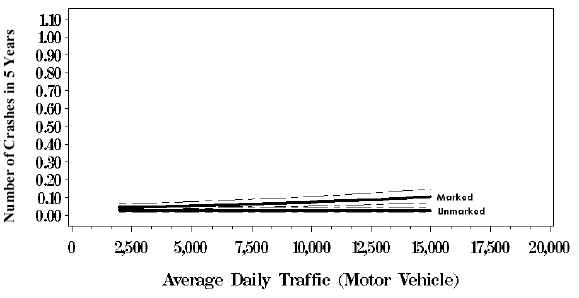Figure 49 Response curves with 95 percent confidence intervals based on negative binomial regression model, two lanes with no median, average daily pedestrian volume = 50.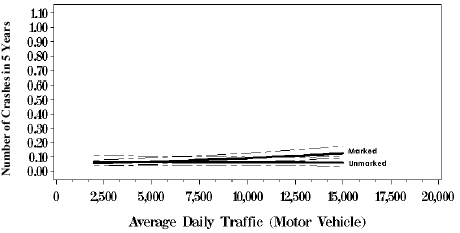Figure 50. Response curves with 95 percent confidence intervals based on negative binomial regression model, two lanes with no median, average daily pedestrian volume = 800.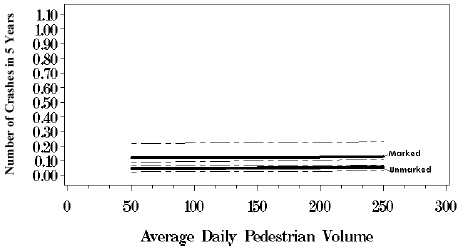Figure 51. Response curves with 95 percent confidence intervals based on negative binomial regression model, five lanes with no median, average daily motor vehicle traffic = 10,000.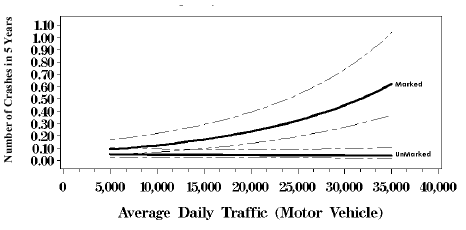Figure 52. Response curves with 95 percent confidence intervals based on negative binomial regression model, five lanes with no median, average daily pedestrian volume = 100.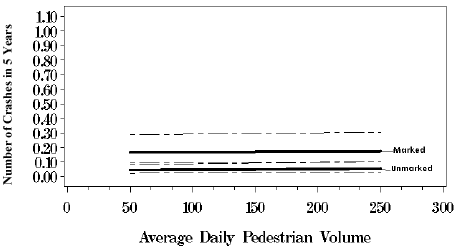Figure 53. Response curves with 95 percent confidence intervals based on negative binomial regression model, five lanes with no median, average daily motor vehicle traffic = 15,000.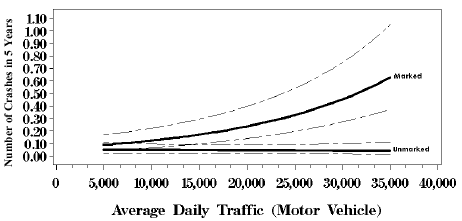Figure 54. Response curves with 95 percent confidence intervals based on negative binomial regression model, five lanes with no median, average daily pedestrian volume = 150.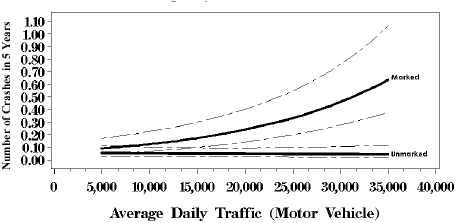Figure 55. Response curves with 95 percent confidence intervals based on negative binomial regression model, five lanes with no median, average daily pedestrian volume = 200.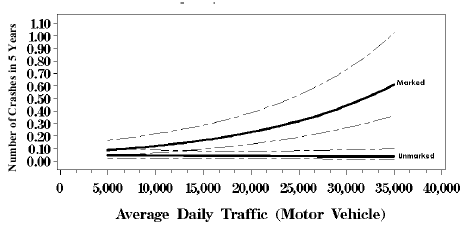Figure 56. Response curves with 95 percent confidence intervals based on negative binomial regression model, five lanes with no median, average daily pedestrian volume = 50.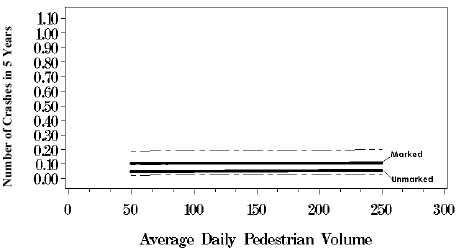Figure 57. Response curves with 95 percent confidence intervals based on negative binomial regression model, five lanes with no median, average daily motor vehicle traffic = 7,500.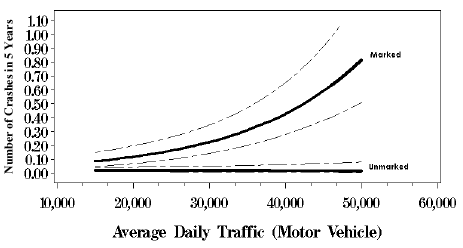Figure 58. Response curves with 95 percent confidence intervals based on negative binomial regression model, five lanes with median, average daily pedestrian volume = 100.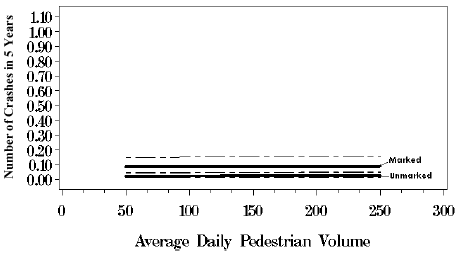Figure 59. Response curves with 95 percent confidence intervals based on negative binomial regression model, five lanes with median, average daily motor vehicle traffic = 15,000.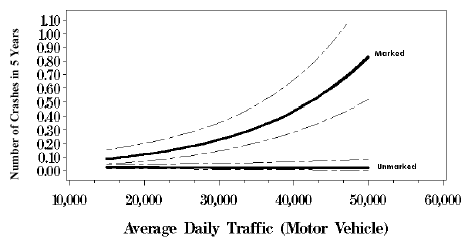Figure 60. Response curves with 95 percent confidence intervals based on negative binomial regression model, five lanes with median, average daily pedestrian volume = 150.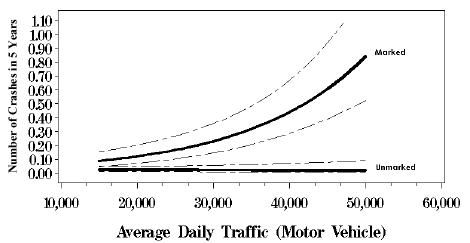Figure 61. Response curves with 95 percent confidence intervals based on negative binomial regression model, five lanes with median, average daily pedestrian volume = 200.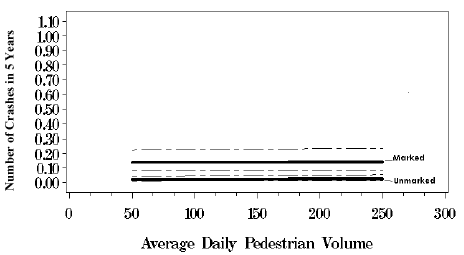Figure 62. Response curves with 95 percent confidence intervals based on negative binomial regression model, five lanes with median, average daily motor vehicle traffic = 22,500.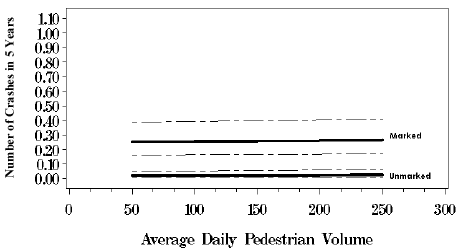Figure 63. Response curves with 95 percent confidence intervals based on negative binomial regression model, five lanes with median, average daily motor vehicle traffic = 32,000.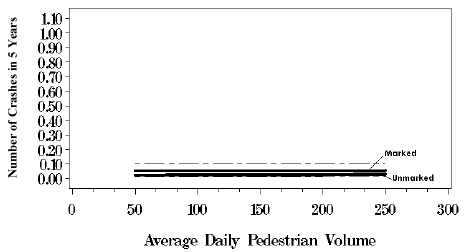Figure 64. Response curves with 95 percent confidence intervals based on negative binomial regression model, five lanes with median, average daily motor vehicle traffic = 7,500.